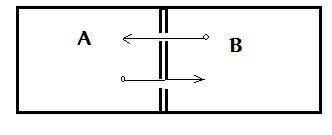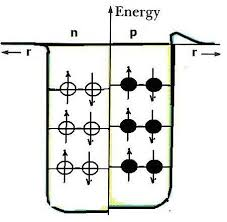## Thursday, November 20, 2014

### An Introduction to Quantum Statistics

1. The Occupancy of States and Distributions:

In statistical physics two particular distributions occupy attention: the Bose-Einstein, and the Fermi-Dirac. For our purposes we will spend much more time on the latter but it is useful to see how both enter the picture.

In the case of the Bose-Einstein formalism we are concerned with bosons which have integral spin values (i.e. an orbital may be populated by any number of bosons so the Pauli Exclusion principle doesn’t apply).

Consider the distribution function for a system of non-interacting bosons in which the system is in

thermal and diffusive contact with a reservoir.  Thus we may allow a circumstance such as this:Fig. 1: Illustrating systems in thermal and diffusive contact.

Thus heat can be exchanged between the systems A and B, as well as particles.  In the formalism treatment we let e denote the energy of a single orbital when occupied by one particle. Hence, when n particles occupy the orbital then the energy must be n e.  To simplify, we treat one orbital as a system and ignore all others.  The grand sum taken over one orbital would then be:

å¥ n = 0   å e   exp ( Nm - e)/ t) =  å¥ n = 0    ln e - n e/t

=  å ¥ n = 0    ( l e - n e/t   )   n

Now, let x =  l e - n e/t     and sum the series in closed form so the grand sum is now:

Ž    å ¥ n = 0    (x) n  =  1/ 1 – x = 1/ [   1 - l e - n e/t ]

Provided:  l e - n e/t       <  1

The ensemble average of the number of particles in the orbital is by definition of the average value:

[ n(e )]  =   å ¥ n = 0    (nx) n  /  å ¥ n = 0    (x) n  =

x (d/dx)  å ¥ n = 0   (x) n / å ¥ n = 0   (x) n  =

x (d/dx) (1x) / (1 – x) -1

After some calculus and messy algebra we find:

[ n(e )]   1/ exp ( em)/ t

Which is the Bose- Einstein distribution.

(N.B. Square brackets used above in place of  R-L 'angle' brackets to prevent problems with blogger)

For the Fermi-Dirac distribution we consider a simplified system represented by a cubical box of volume V = L 3

The number of electrons (Fermi particles) within occurs between energies e and e + de.  The energies occur in a quantized way and are depicted schematically below:Fig 2. Box with Fermi occupancy and energy levels.

The diagram shows each level corresponding to two orbitals, one for spin up the other for spin down.

The Fermi energy:

e F = ħ( p n F / L) 2 /2m

Is determined by the requirement that the system in the ground state holds N electrons – with each orbital filled with one electron if the energy of the orbital is less than:
e F.

Since for a 3D box the quantized energy is:

ħ2/2m [p n2 / L]

where n2 =  n x 2  +  n y 2    + n z

Then the number of states possessed by a 3D box is:

1/8 [4p n3 / 3]

For a sphere, the number of orbitals in some radius is:

n=  g  [4p n3 / 3]  (1/8) =  g p n3  / 6

For electrons,  g   = 2 so:   n=  p n3  / 3

Hence, if the box holds N electrons the orbitals must be filled up to the quantum number n F.    So the total number of electrons is:

N =    p n F/ 3  so     n F  = (3N/p) 1/3

The Fermi energy would then be:

e F = ħ( p / L) n F 2/ 2m   =

ħ( p / L) (3N/p) 1/3/ 2m

Since  V = L 3 we can write:

e F = ħ/ 2m   [3p2 N/ v] 2/3

This yields good results for monovalent atoms because they have only one electron per atom.

2. Treating the Nucleus as a Fermi Gas

One of the useful applications of the Fermi quantum statistics is to the atomic nucleus, such as that for the deuteron.  The key to the application is assuming an independent particle model so each nucleon moves in a smooth potential hole as opposed to being subject to the actions of (A- 1) other nucleons.  In this sense, the model would exhibit similar properties to the “electron gas” which we examined being confined to a 3D box in the previous section.  In this case, the nucleons will be constrained to move in a spherical potential hole of radius:

R = r o A 1/3

(I.e. analogous to the case of electrons)

We focus then on all momentum states being filled up to the Fermi momentum,   p F.

Since:   p F 2/ 2m  =  ħ / 2m   [3p2 N/ v] 2/3

the number of states up to  p F  per unit volume is:

N/ v  =    4p/ 3  ( p F 3/ h3 )

Or the volume in momentum space divided by the Planck constant. Let’s now apply this to the case of the deuteron, for which we have a neutron and proton in each state and each can have spin up or spin down. Then we get a total of four states so multiply  the expression by 4 to get:

4N =  4[4p/ 3 ( c h3 ) (4p R 3/ (2 p h3 ) 3]

Simplifying:

p F r o / h =    Ö (9 p/ 8)  =   1.52

This prescribes:  the maximum momentum present in the nucleus in terms of the nuclear radius  r o .
p F  =    1.52 (h/ r o)

Taking r o =   1.1 fm (e.g. 1.1 x 10 -15 m)

We learn the maximum kinetic energies in the nucleus are as large as:

K F  =    p F 2/ 2m  =  39 MeV

If the binding energy of the ‘last nucleon’, e.g. at the top of the Fermi distribution) is » 8 MeV then this result shows that the nucleus looks to each nucleon (say to the neutron or proton  in Deuterium) like a potential hole of 39 MeV depth.

Suggested Problems:

1) Find the Fermi sphere parameters: e F ,  v F  and   T F for He3 at absolute zero, viewed as a gas of non-interactive fermions. (The density of the liquid is 81 kg/ m 3).

2) a) Show that (- f / e)  evaluated at the Fermi level (e  = m) has the value (4 kB T) -1. Thus, the lower the temperature, then the steeper the slope of the Fermi-Dirac function.

Hint: Use f(e=  1/ [exp  (e- m )/ t + 1]

b) Make a careful plot of   (- f / e) vs.  e/ kB  for  the specific cases:

m/ kB   =  5 x 10 4 K  and  5 x 10 2 K .

3) Let emd,  show that: f(d  ) = 1 – f( -d )

Hint: Let f(d  ) =  1/ [exp (m - e)/ t + 1]

4) Treating the Tritium nucleus as a nucleonic electron gas, assume an independent particle model (based on Fermi energy levels)  so each nucleon moves in a smooth potential hole.
Thereby obtain the maximum momentum present in the nucleus in terms of the nuclear radius  r o .

p F  =    1.52 (h/ r o)

(Take r o =   1.1  fm )

Use this to estimate the depth of the potential hole, i.e. the magnitude of the well in MeV for how the nucleus looks to each nucleon (say to the neutrons or proton in Tritium) .

Give a general idea of how errors enter your estimate and explain why it can’t be taken too literally.